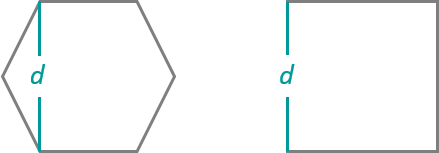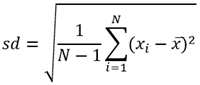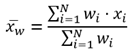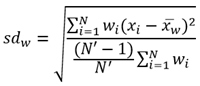# 范围内汇总

##### 注：“范围内汇总”工具可计算输入图层与边界图层重叠区域的统计数据。

## 工作流示意图## 示例

• 某城市面临维护工程的积压问题，并希望将维护评估地区融入与街灯和自行车车道维护相关的项目中。 范围内汇总工具用于计算每个地区的街灯数和自行车道的英里数，以便有效安排工程的优先级。

• 一家有线电视提供商即将启动一项试点工程，该工程可使收入较低的社区大学的学生以较低的成本访问互联网。 范围内汇总工具用于确定各学区内低收入家庭的数量，以便有线电视提供商可以选择合适的地区来实施其试点工程。

• 一家开发公司正在考虑在城区中心打造综合性开发项目。 该公司可以使用范围内汇总来汇总城市边界内具有资格的区域，以获得潜在开发区的总范围。

## 用法说明• 英里
• 英尺
• 千米

Areas

• 平方英里
• 平方千米
• 平方米
• 公顷
• 英亩

## 局限性

• 边界输入必须是面要素。
• 线和面是按比例进行汇总的；汇总线或面时，最好汇总绝对数据（如人口）而非相对数据（如平均收入）。
• 无法选择移除与任何点、线或面都不重叠的边界。

## 范围内汇总工作原理

### 等式• N = 观测值个数
• xi = 观测值
• = 平均数• N = 观测值个数
• xi = 观测值
• wi = 权重• N = 观测值个数
• xi = 观测值
• wi = 权重
• w = 加权平均数
• N' = 非零权重数

### 点

``280 + 408 + 356 + 361 + 450 + 713 = 2,568``

``[280, 408, 356, 361, 450, 713] = 280``

``[280, 408, 356, 361, 450, 713] = 713``

``2,568/6 = 428````= 150.79``

### 线

``400 + 500 = 900``

``[400, 500] = 400``

``[400, 500] = 500````= 460````= 69.28``

### Areas

``2,133 + 3,133 + 400 + 3,375 + 1,800 = 10,841``

``[2,133, 3,133, 400, 3,375, 1,800] = 400``

``[2,133, 3,133, 400, 3,375, 1,800] = 3,375````= 2,665.53````= 925.91``